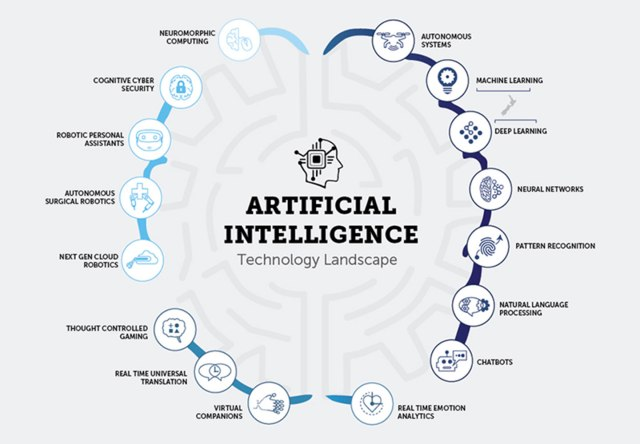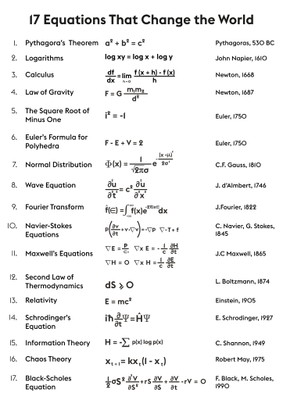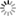##### Personal tools
You are here: Home Mathematics for AI/ML/DL, OR/MS/IE, and Data Science

# Mathematics for AI/ML/DL, OR/MS/IE, and Data Science[AI Technology Landscape - Uni-part Security]

### - Overview

Mathematics is an important aspect of machine learning. While some people may absolutely love math, others may not. However, one must have at least some mathematical knowledge and understand the concepts of probability, statistics, and calculus to successfully solve machine learning tasks. You can't do anything without math. Everything around you is math. Everything around you is digital.

The main branches of mathematics involved in artificial intelligence are: Calculus, linear algebra, probability, and statistics. Linear Algebra is the field of applied mathematics which is something AI experts can't live without. You will never become a good AI specialist without mastering this field.

The relationship between AI and mathematics can be summarized as:

• A person in AI who doesn't know math is like a politician who doesn't know how to persuade. Both have an unavoidable field of work!
• A popular suggestion for learning math for AI is this: learn linear algebra, probability, multivariate calculus, optimization, and a few other topics.

### - The Mathematics Involved in AI/ML/DL, Neural Networks, and Data Science

Driven by data, machine learning (ML) models are the mathematical engines of artificial intelligence, algorithmic expressions that can discover patterns and make predictions faster than humans. For the most transformative technological AI journey of our time, the engine you need is a machine learning model.

For example, an ML model for computer vision might be able to identify cars and pedestrians in live video. A translatable word and sentence for natural language processing.

However, math can be daunting, especially for someone from a non-technical background. Apply this complexity to machine learning and you're in a very bad place. We can easily build models and perform various machine learning tasks using widely available libraries in Python and R. So it's easy to avoid the math part of the field.[The 17 equations that changed the world]

### - Mathematics is the Mother of All Sciences

Mathematics is considered the mother of all sciences as it is the tool for solving problems in all other sciences. Mathematics applied in all fields of science, including physics, engineering, biology and economics. Mathematics also helps develop critical thinking, problem-solving ability and logical reasoning ability.

Emmanuel Kant said: "A science is only accurate if it uses mathematics." Carl Friedrich Gauss called mathematics the "queen of science" because of its great success in revealing the nature of physical reality.

However, some people believe that mathematics is not a science. They say that science is devoted to understanding the physical world, and that our senses are the only way we receive information about the physical world. Mathematics, on the other hand, is meaning-agnostic.

Mathematics (from Greek μάθημα máthēma “knowledge, study, learning”) is the study of quantity, structure, space, and change. Mathematicians seek out patterns and formulate new conjectures. Mathematicians resolve the truth or falsity of conjectures by mathematical proof. The research required to solve mathematical problems can take years or even centuries of sustained inquiry.

Since the pioneering work of Giuseppe Peano (1858-1932), David Hilbert (1862-1943), and others on axiomatic systems in the late 19th century, it has become customary to view mathematical research as establishing truth by rigorous deduction from appropriately chosen axioms and definitions.

When those mathematical structures are good models of real phenomena, then mathematical reasoning often provides insight or predictions.

[More to come ...]

##### Document Actions